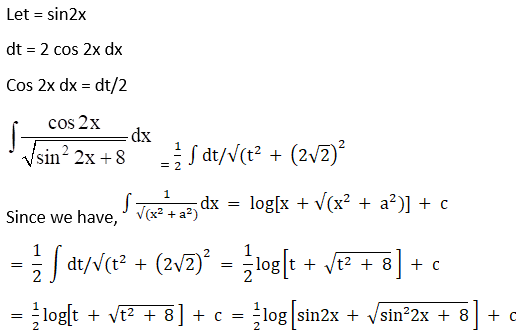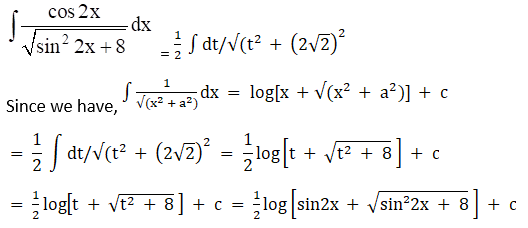# RD Sharma Solutions For Class 12 Maths Exercise 19.18 Chapter 19 Indefinite Integrals

Students having any doubts on the concepts of Class 12 RD Sharma textbooks can quickly refer to the RD Sharma Solutions for Class 12, as per their convenience. This is the best resource for any reference and helps students prepare confidently for their examinations. This exercise deals with determining the integrals reducible to the form $\int \frac{1}{\sqrt{ax^{2} + bx + c}} \ dx$ along with algorithm. The exercise problems of Chapter 19 are solved by a very well experienced faculty having in-depth knowledge about these concepts. In addition, the solutions are prepared, as per the weightage allotted in the board exam.

## Download the PDF of RD Sharma Solutions For Class 12 Chapter 19 – Indefinite Integrals Exercise 19.18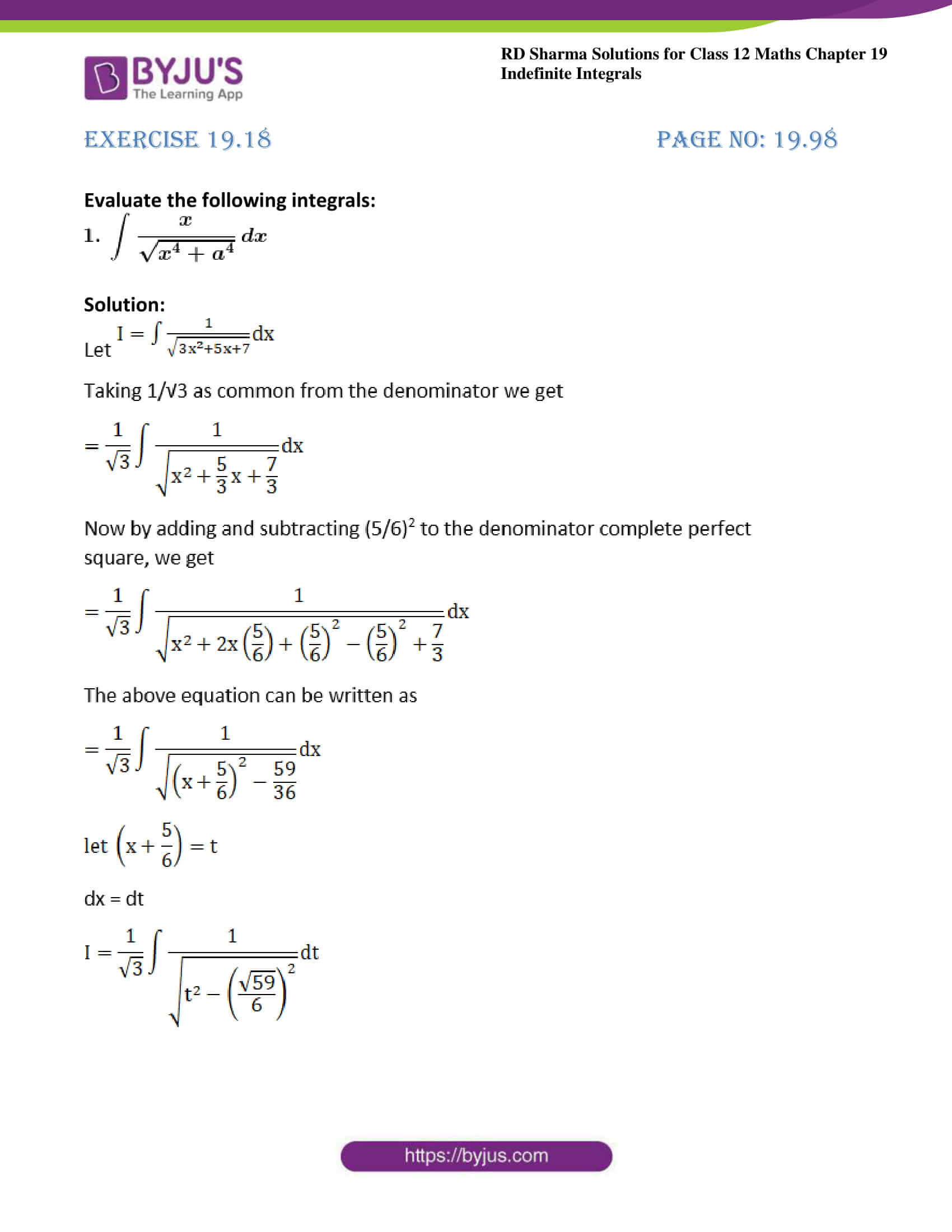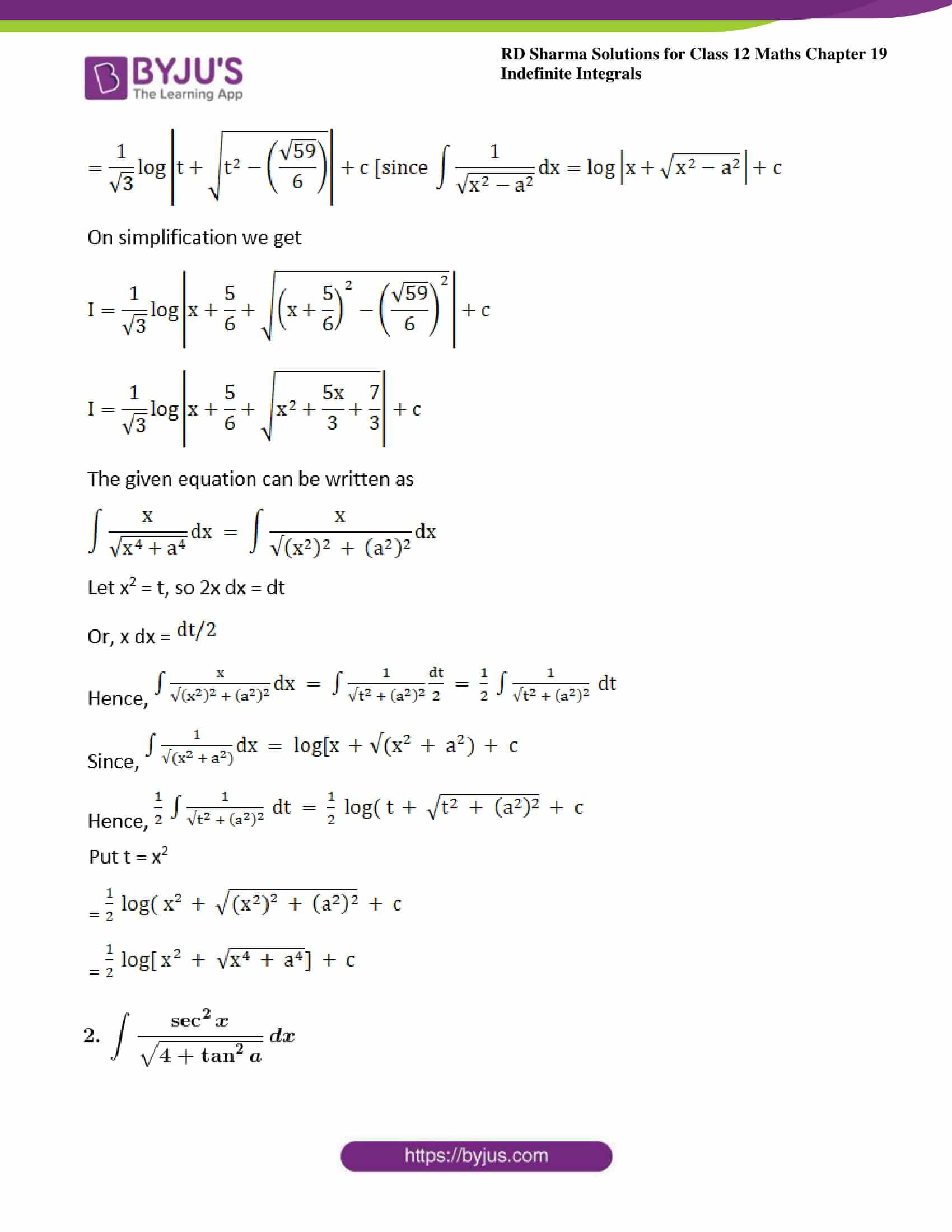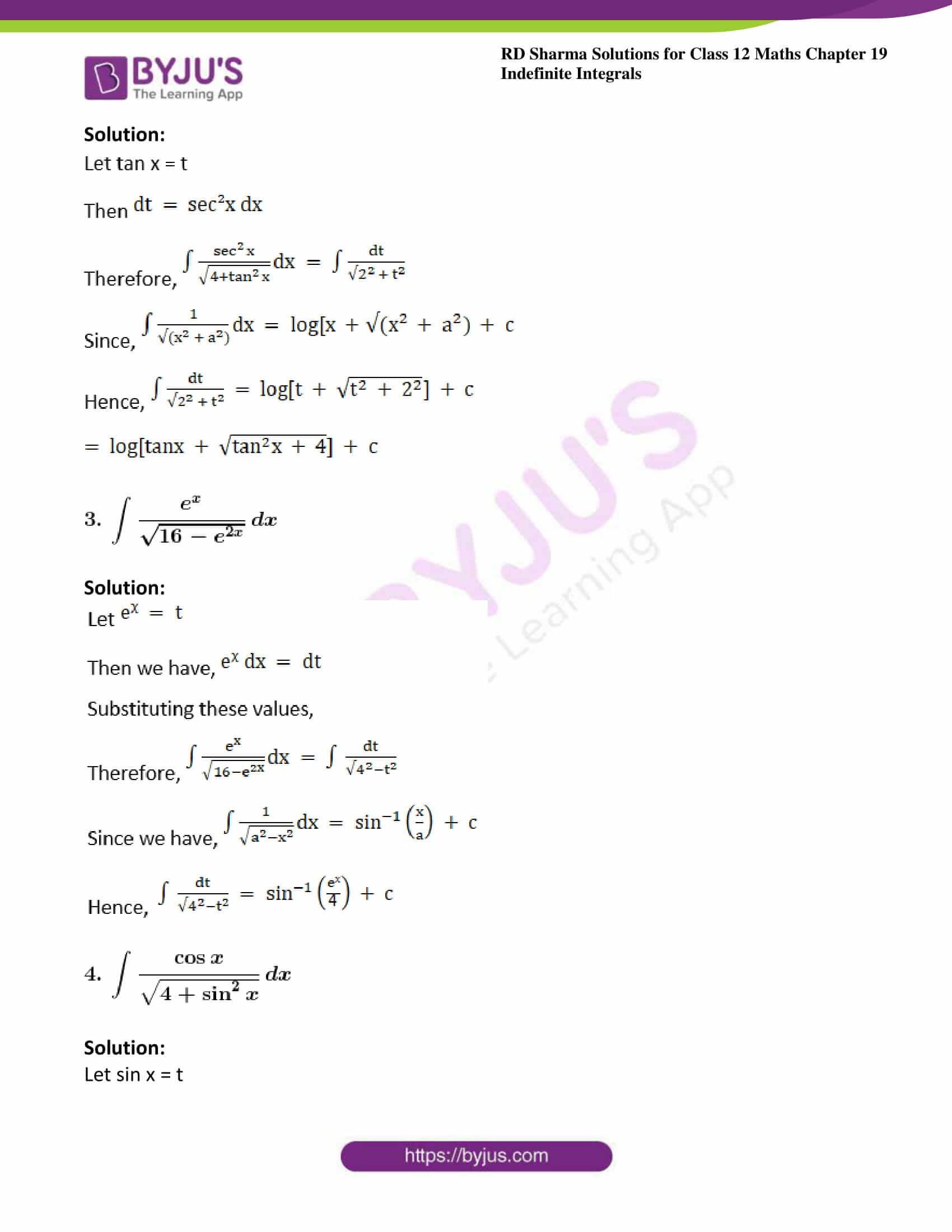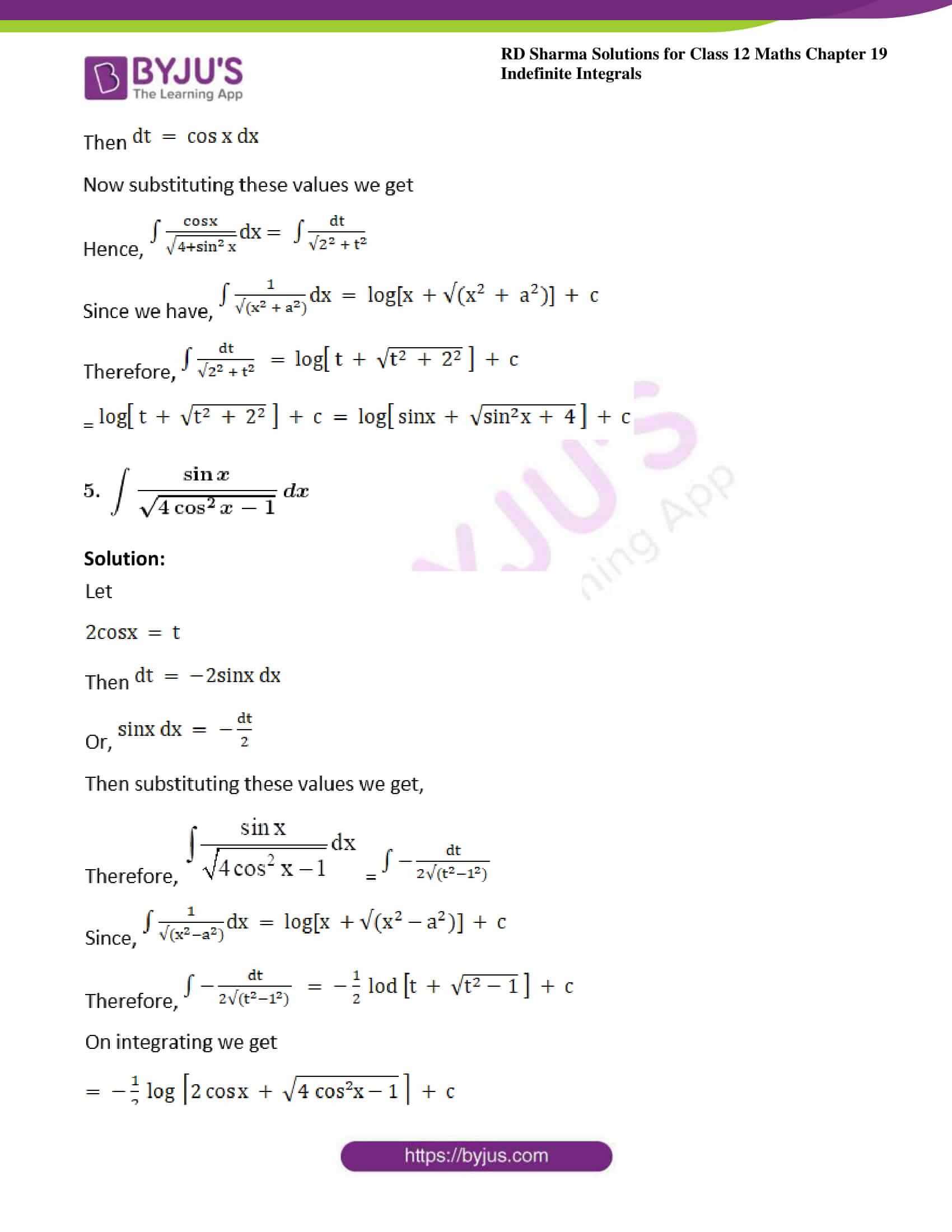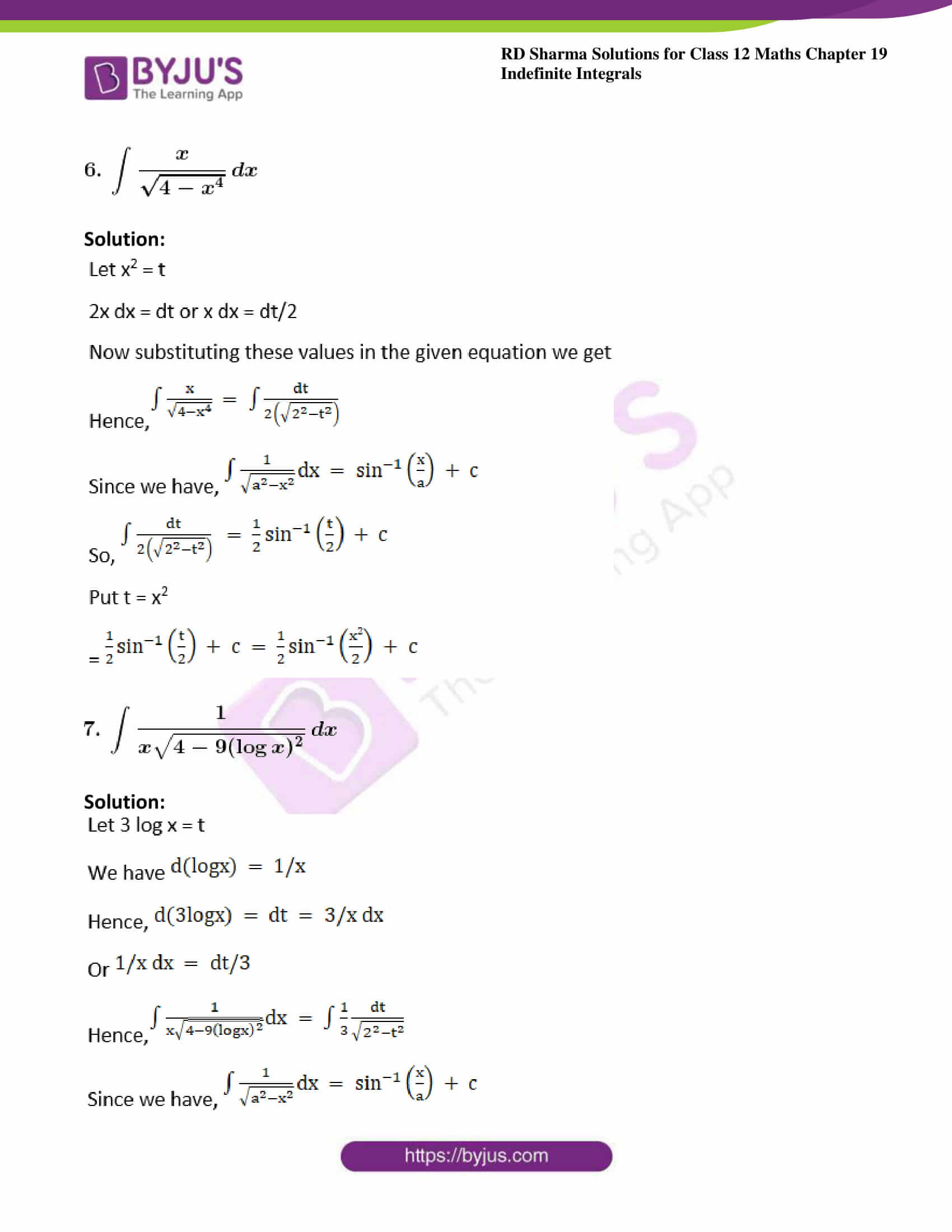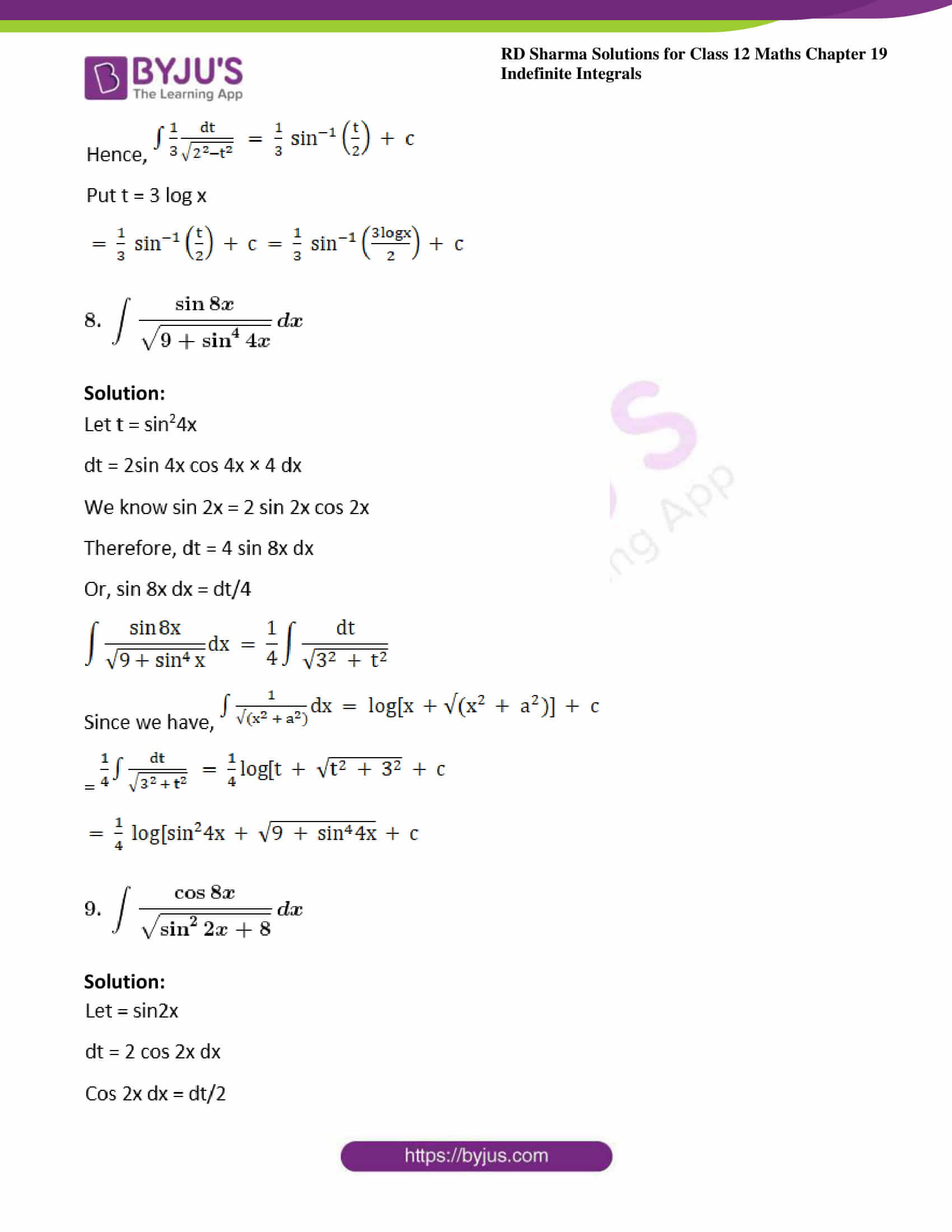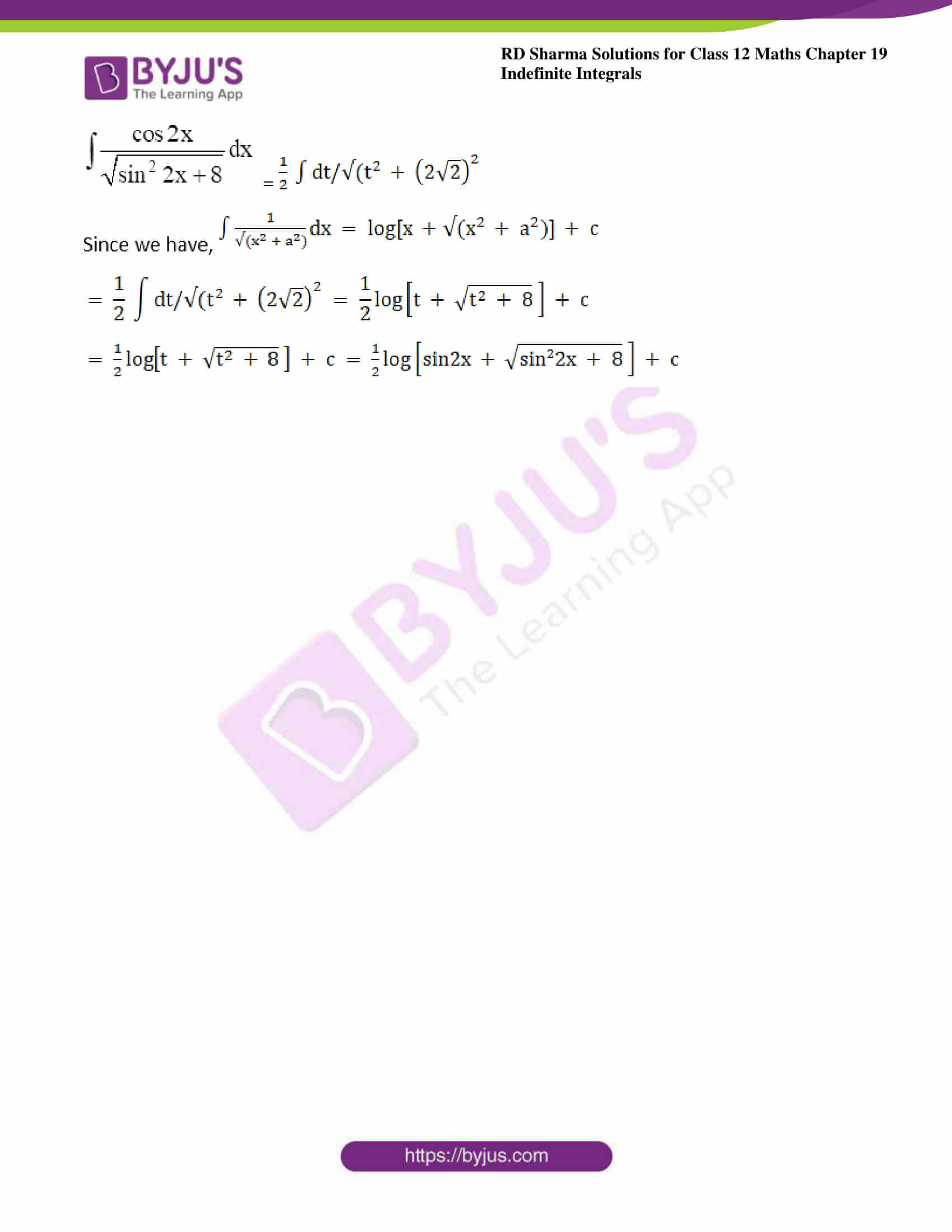### Access answers to Maths RD Sharma Solutions For Class 12 Chapter 19 – Indefinite Integrals Exercise 19.18

Evaluate the following integrals: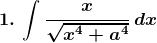Solution: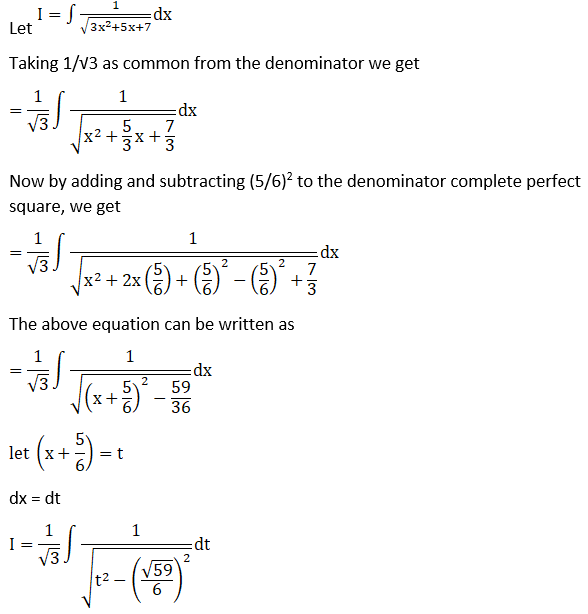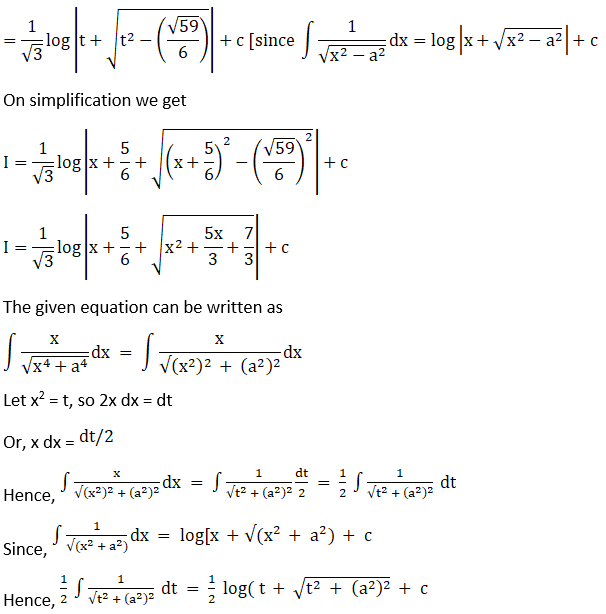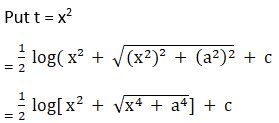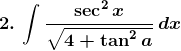Solution: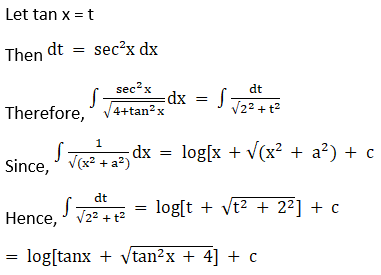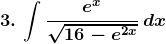Solution: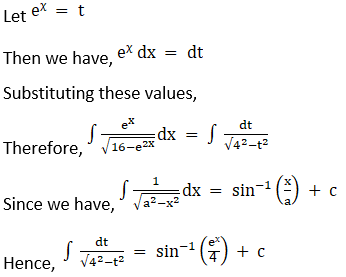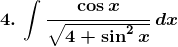Solution:

Let sin x = t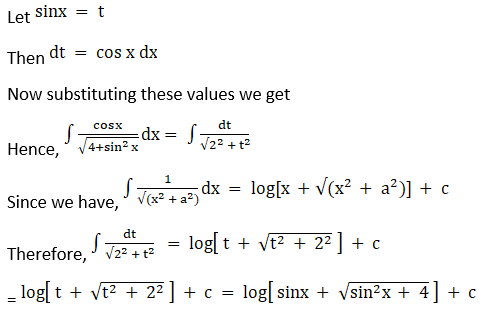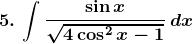Solution: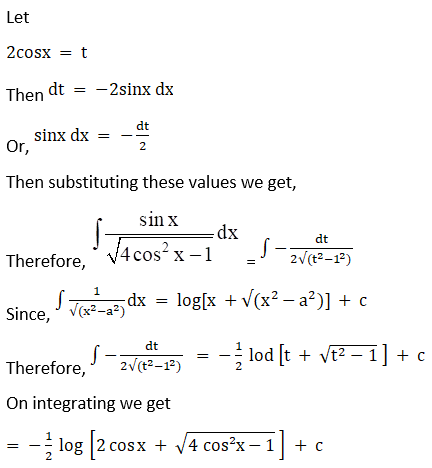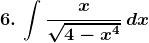Solution: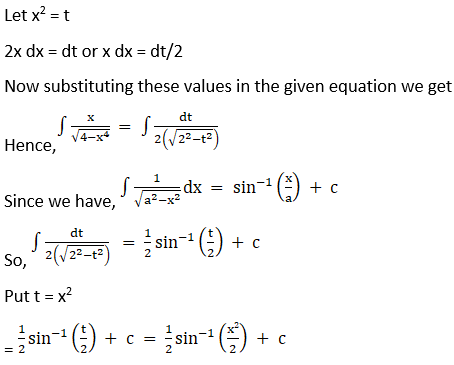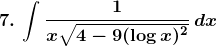Solution: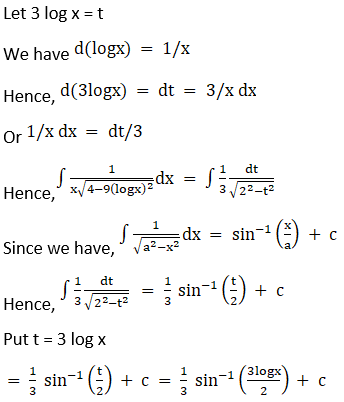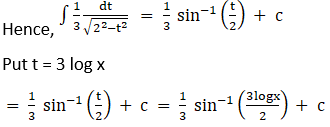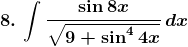Solution: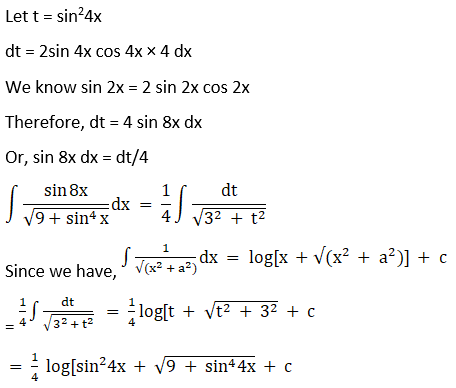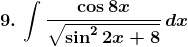Solution: